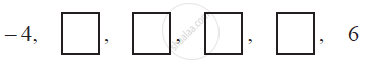# In the following APs, find the missing terms in the boxes - Mathematics

In the following APs, find the missing terms in the boxes :#### Solution

For this A.P.,

a = −4 and

a6 = 6

We know that,

an = a + (n − 1) d

a6 = a + (6 − 1) d

6 = − 4 + 5d

10 = 5d

d = 2

a2 = a + d = − 4 + 2 = −2

a3 = a + 2d = − 4 + 2 (2) = 0

a4 = a + 3d = − 4 + 3 (2) = 2

a5 = a + 4d = − 4 + 4 (2) = 4

Therefore, the missing terms are −2, 0, 2, and 4 respectively.

Concept: nth Term of an AP
Is there an error in this question or solution?

#### APPEARS IN

NCERT Class 10 Maths
Chapter 5 Arithmetic Progressions
Exercise 5.2 | Q 3.4 | Page 106